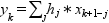Scilab Home page | Wiki | Bug tracker | Forge | Mailing list archives | ATOMS | File exchange
Change language to: Français - Português - 日本語

See the recommended documentation of this function

Scilab help >> Signal Processing > convol

convol

convolution

Calling Sequence

```[y]=convol(h,x)
[y,e1]=convol(h,x,e0)```

Arguments

h

a vector, first input sequence ("short" one)

x

a vector, second input sequence ( "long" one)

e0

a vector,old tail to overlap add (not used in first call)

y

a vector, the convolution.

e1

new tail to overlap add (not used in last call)

Description

Calculates the convolution `y= h*x` of two discrete sequences by using the fft. The convolution is defined as follow:Overlap add method can be used.

USE OF OVERLAP ADD METHOD: For `x=[x1,x2,...,xNm1,xN]` First call is `[y1,e1]=convol(h,x1);` Subsequent calls : `[yk,ek]=convol(h,xk,ekm1)`; Final call : `[yN]=convol(h,xN,eNm1);` Finally `y=[y1,y2,...,yNm1,yN]`.

The algorithm based on the convolution definition is implemented for polynomial product: `y=convol(h,x)` is equivalent to `y=coeff(poly(h,'z','c')*poly(x,'z','c')` but much more efficient if `x` is a "long" array.

Examples

```x=1:3;
h1=[1,0,0,0,0];h2=[0,1,0,0,0];h3=[0,0,1,0,0];
x1=convol(h1,x),x2=convol(h2,x),x3=convol(h3,x),
convol(h1+h2+h3,x)
p1=poly(x,'x','coeff')
p2=poly(h1+h2+h3,'x','coeff')
p1*p2```

• corr — correlation, covariance
• fft — fast Fourier transform.
• pspect — two sided cross-spectral estimate between 2 discrete time signals using the Welch's average periodogram method.

Authors

F. D , C. Bunks Date 3 Oct. 1988; ;